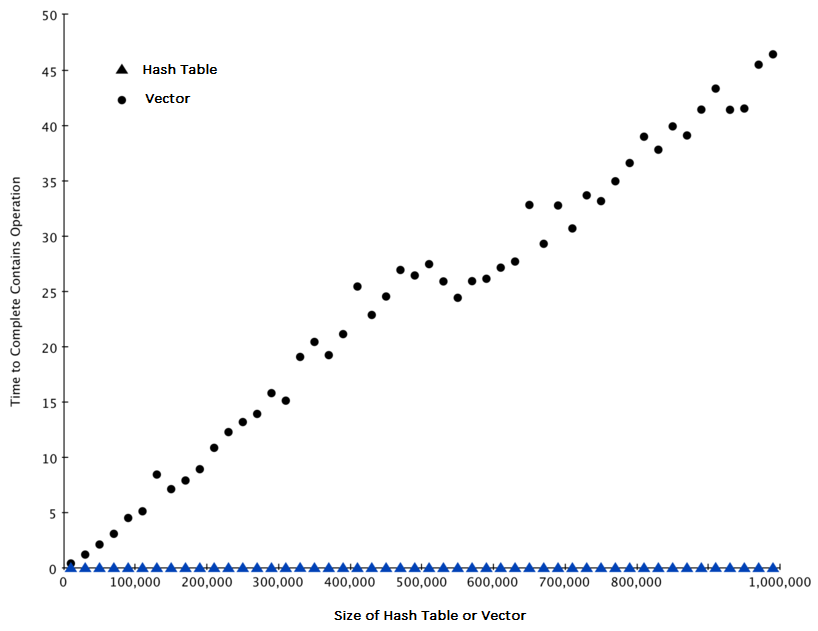# 2.8. Analysis of Hash Tables¶

The second major C++ data structure to analyze is the hash table or hash map. As you may recall, hash tables differ from vectors in that you access items in a hash table by a key rather than a position. A hash table (hash map) is a data structure that maps keys to their associated values using a hash function. The underlying structure is a mostly empty array, but unlike the array or vector data structures, the hash table values are not stored contiguously. The hash function computes an index into the array of “buckets” from which the correct associated value can be located. Ideally, the hash table values are distributed uniformly in the underlying array and, again ideally, the hash function will assign each key to a unique bucket. However, most hash tables are designed to handle hash collisions when the hash function generates the same bucket index for more than one key. More about this when we discuss implementations in more depth.

Later in this book you will see that there are many ways to implement a hash table. The thing that is most important to note now is that the get item and set item operations on a hash table are $$O(1)$$. Another important hash table operation is the contains operation. Unlike a vector, checking to see whether a value is in the hash table or not is also $$O(1)$$.

The efficiency of all hash table operations is summarized in Table 6. One important side note on hash table performance is that the efficiencies we provide in the table below are for average performance. In some rare cases the contains, get item, and set item operations can degenerate into $$O(n)$$ performance but we will get into that in a later chapter when we talk about the different ways that a hash table could be implemented.

Table 4: Big-O Efficiency of C++ hash table Operations

operation

Big-O Efficiency

find

O(1)

insert

O(1)

erase

O(1)

contains

O(1)

iteration

O(n)

Note that it is not typical to iterate through a hash table because it is a data structure designed for look-up, not for iteration. The big advantage of the hash table is the constant speed of the contains operation.

For our last performance experiment we will compare the performance of the contains operation between vectors and hash tables. In the process we will confirm that the contains operator for vectors is $$O(n)$$ and the contains operator for hash tables is $$O(1)$$. The experiment we will use to compare the two is simple. We’ll make a vector with a range of numbers in it. Then we will pick numbers at random and check to see if the numbers are in the vector. If our performance tables are correct the bigger the vector the longer it should take to determine if any one number is contained in the vector.

We will repeat the same experiment for a hash table that contains numbers as the keys. In this experiment we should see that determining whether or not a number is in the hash table is not only much faster, but the time it takes to check should remain constant even as the hash table grows larger.

Listing 6 implements this comparison. Notice that we are performing exactly the same operation, number in container. The difference is that on line 7 x is vector, and on line 9 x is a hash table.

Listing 6

#include <iostream>
#include <ctime>
#include <vector>
#include <unordered_map>
using namespace std;

int main() {

for(int a = 10000; a < 1000001; a = a + 20000) {
// vector Part
clock_t begin = clock();
vector<int> avector;
for( int i = 0; i < a; i++){
avector.push_back(i);
}
clock_t end = clock();
double elapsed_secs = double(end - begin) / CLOCKS_PER_SEC;

// Hash Table Part
clock_t  begin_ht = clock();
unordered_map<int, int> y;
for( int j = 0; j < a; j++ ){
y[j] = NULL;
}
clock_t end_ht = clock();
double elapsed_secs_ht = double(end_ht - begin_ht) / CLOCKS_PER_SEC;

// Printing final output
cout << a << "\t" << elapsed_secs << "\t" << elapsed_secs_ht << endl;
}

return 0;
}

 1import timeit
2import random
3
4for i in range(10000,1000001,20000):
5    t = timeit.Timer("random.randrange(%d) in x"%i,
6                     "from __main__ import random,x")
7    x = list(range(i))
8    lst_time = t.timeit(number=1000)
9    x = {j:None for j in range(i)}
10    d_time = t.timeit(number=1000)
11    print("%d,%10.3f,%10.3f" % (i, lst_time, d_time))


Figure 3 summarizes the results of running Listing 6. You can see that the hash table is consistently faster. For the smallest vector size of 10,000 elements a hash table is 89.4 times faster than a vector. For the largest vector size of 990,000 elements the hash table is 11,603 times faster! You can also see that the time it takes for the contains operator on the vector grows linearly with the size of the vector. This verifies the assertion that the contains operator on a vector is $$O(n)$$. It can also be seen that the time for the contains operator on a hash table is constant even as the hash table size grows. In fact for a hash table size of 10,000 the contains operation took 0.004 milliseconds and for the hash table size of 990,000 it also took 0.004 milliseconds.Figure 3: Comparing the in Operator for C++ vectors and Hash Tables¶

Since C++ is an evolving language, there are always changes going on behind the scenes. The latest information on the performance of C++ data structures can be found on the C++ website.

Self Check

# 2.9. Summary¶

• Algorithm analysis is an implementation-independent way of measuring an algorithm.

• Big-O notation allows algorithms to be classified by their dominant process with respect to the size of the problem.